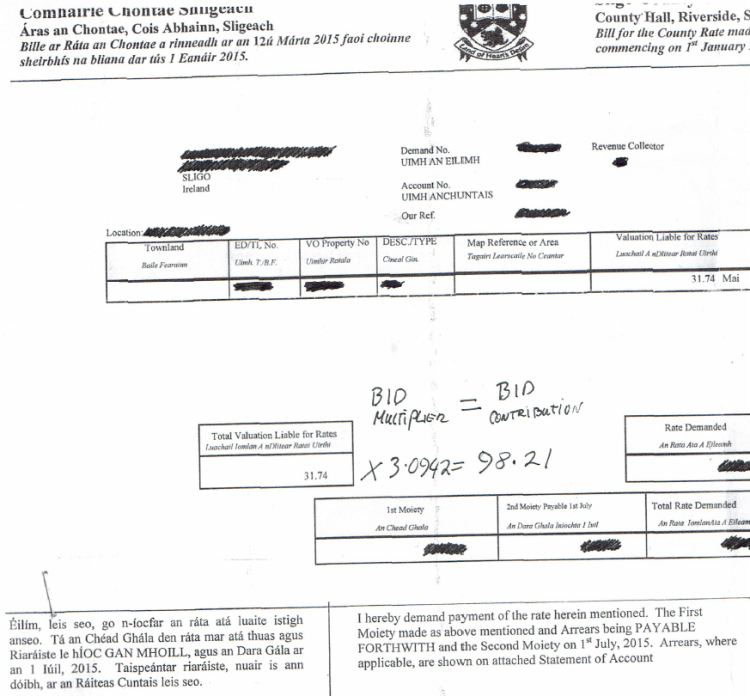# Bid Contribution

The amount if calculated by the Valuation Liable for Rates x BID Multiplier (3.0942) = BID Contribution.In this example: the Rates valuation = €31.74 x 3.0942 (BID Multiplier) = €98.21 p.a.